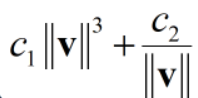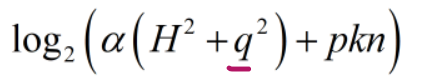SOS!!! I don’t know how to use pow_abs and log function with {convex}. I have a very weak foundation here. Can you give me the solution and some related web pages?
(1). pow_abs with cvx

The original formula:(2). And ‘log’ function use rel_entr function.

The original formula:Use `pow_pos(norm(...),3)` instead of `pow_abs(norm(...),3)` for `norm(...)^3`.

`1/norm(v)` is neither convex nor concave. For instance, if v1) = v(2) = 1, its Hessian has one positive and one negative eigenvalue.

The log term is going to switch between convex and concave depending on the value of q^2.